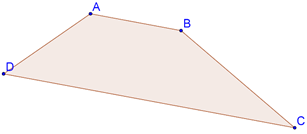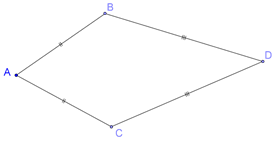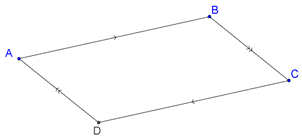# Some Particular Types of Quadrilaterals

Go back to  'Triangles-Quadrilaterals'

## Trapezium

A trapezium is a quadrilateral in which one pair of opposite sides is parallel. In the following figure, ABCD is a trapezium since AB || CD:## Kite

A kite is a quadrilateral in which two pairs of adjacent sides are equal. In the following figure, ABCD is a kite, as AB = AC and DB = DC:## Parallelogram

A parallelogram is a quadrilateral in which both pairs of opposite sides are parallel. In the following figure, ABCD is a parallelogram as AB || CD and AD || BC: